## Saturday, January 5, 2013

### Growing Squares and Circles inside Squares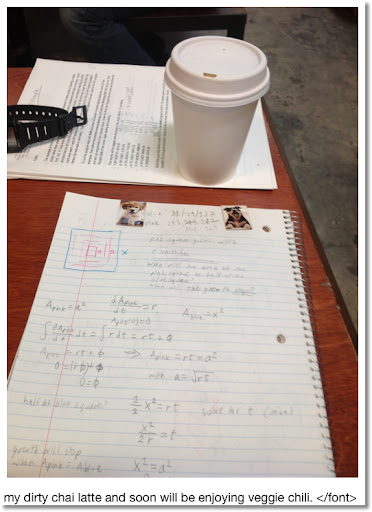Happy New Year everyone! I am blogging from Stories Books and Cafè in Los Angeles. Today's blog is about geometry mixed a little calculus. Let's have fun!

Growing a Square inside a Square

Imagine a blue square with length x. Then from the center of the blue square, a pink square grows at a constant rate r. How long will it take for the pink square to grow as big as the blue square?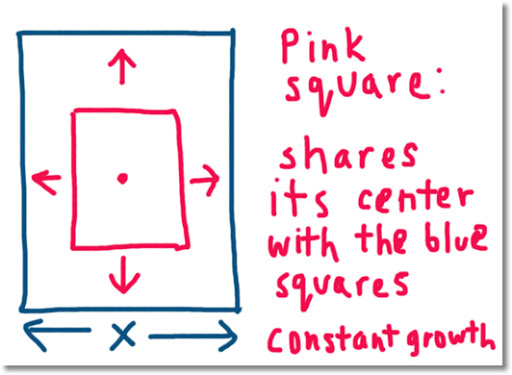Let a = the length of pink's square side. If A represents the area of a square, then let:

Ab = area of the blue square = x² and
Ap = area of the pink square = a².

Note Ap is also a function of time, t, as Ap is changing.

The growth of the pink square can be described as:

dAp/dt = r with the initial condition Ap(0) = 0. Here I assume that the pink square starts out so small it's initial area is negligible.

Then:

∫ dAp/dt dt = ∫ r dt
Ap(t) = r * t + C, for constant C.

Considering the initial condition Ap(0) = 0, implies that C = 0, and Ap(t) = r * t.

So how long does it take for the pink square to grow as big as the blue square? Simply, this happens when the areas of both blue and pink squares equal.

Ab = Ap
x² = r * t
⇒ t = x²/r

For example, if the length of the blue square is x = 5 inches and the rate of the pink square grows at r = 0.5 in/sec, then it takes 5²/0.5 = 50 seconds for the pink square to grow as big as the pink square.

Two Squares Growing

Now let's consider two squares that start out as dots (with virtually no initial area of their own). Start with our blue square with length x and area x^2.

Then place one square, let it be purple and label it A with its center located at (x/4, x/2). Place another square, let it be pink and label it B with its center located at (3x/4, x/2).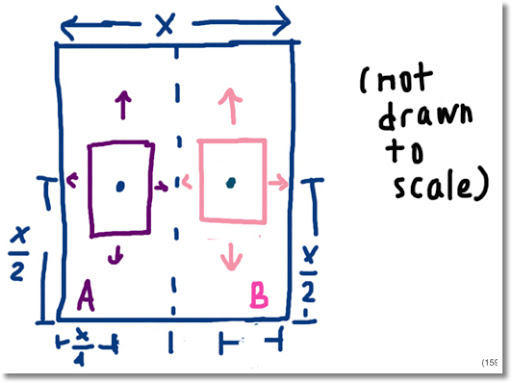Assume the following:

1. The growth of both the purple and pink square is constant, r.

2. The purple and pink squares stop growing when they touch each other.

3. The growth of both squares start simultaneously.

Let:
S = Area of the blue square = x²
A = Area of the purple square
B = Area of the pink square

Both A and B are functions of time, t since they change. Then:

dA/dt = r
∫ dA/dt dt = ∫ r dt
A = r * t + C

Considering the initial condition A(0) = 0, A = r * t.

Likewise, B = r * t.

When do the purple and pink squares meet? Inferring from the picture above, both squares meet when the length of each square reaches the length x/2. Since both squares grow at the same time, we can find out the time for each square by considering only one of the two squares. Without loss of generality, consider the purple square.

The maximum area of A is x²/4. Since A = r * t,

x²/4 = r * t
⇒ t = x²/(4*r)

How big will the two squares get? Considering the maximum size of the purple (and pink) square is x²/4, the largest the two squares will grow (without intersection) is:

x²/4 + x²/4 = x²/2

Growth of a Circle inside of a Square at a Constant Rate

Now consider a circle with radius r with its center located at the center of a square (x/2, x/2). Consider the growth of the circle constant, at rate α. The circle starts as a dot with virtually no area.

How long will it take until the circle grows as big as it can inside the square?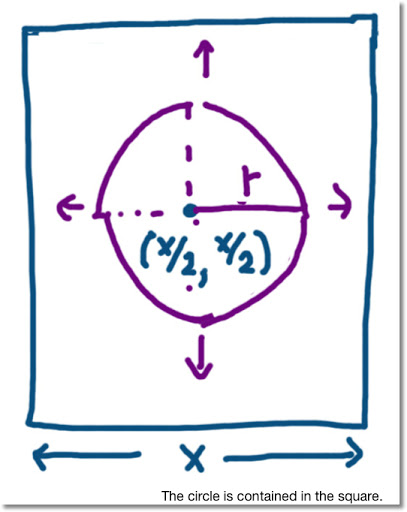The circle will touch the square when its radius extends from the center of the square to one of its edges. But where?

Consider the measure of the radius as a line of coming from the center of the square and growing in a direction parallel to any on of its sides ("up", "down", "left", or "right"). (Hopefully this makes sense). For the moment, allow the circle to grow only when it's entire shape lies inside the square. Then the circle gets to its largest shape when r = x/2.

When will that happen?

Let A be the area of the circle, so A = π * r² and dA/dt = α.

With the initial condition A(0) = 0, A = α * t.

With r = x/2,

α * t = (x/2)² * π
α * t = (x² * π)/4
⇒ t = (x² * π)/(4 * α)

How big is the largest circle? At the largest radius, A = (x² * π)/4

The area of the square is x². Clearly, the area of the square is larger than the circle. Taking a ratio of the circle's area to the square's area:

((x² * π)/4) / x² = π /4 ≈ 0.78540

The largest circle that can be completely contained inside the square has an area that is about 78.5% of the square's area. Cool huh?

What if the Circle is allowed to grow beyond the Square's Borders?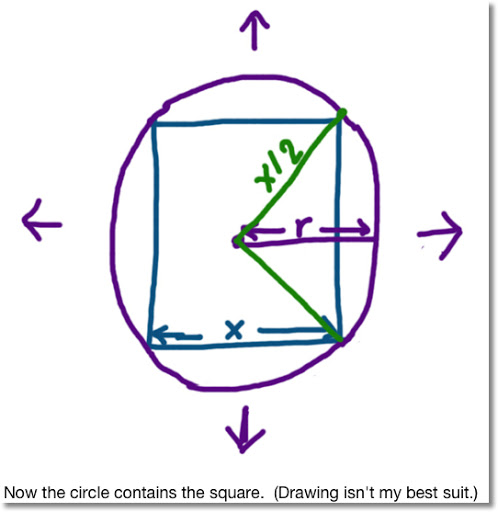At what point does the circle completely encompass the square? In other words, at what point does the circle start containing the square?

This happens with the square's corners, and only its corners touches the circle. Let's determine the area of the circle at this point.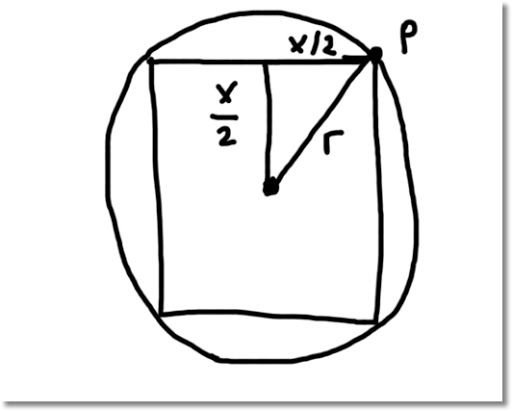Consider the point P in the above diagram. P is also on one of the square's corners. Since the center of the circle and the center of the square are at the same location, the length from point P to the center is r.

Then by the Pythagorean Theorem:

r^2 = (x/2)^2 + (x/2)^2
⇒ r = x/√2

Then the circle's area is A = x^2 * π * 1/2 and the time it takes for the circle to get that large is t = (x^2 * π)/(2 * α).

At this point the area of the circle is π/2, or about 1.57080 times the area of the square.

By the way, the chili was spicy, and good. Thanks and stay safe! Eddie

This blog is property of Edward Shore. 2013

### Retro Review: Radio Shack EC-4026

Retro Review:   Radio Shack EC-4026 Quick Facts: Model:  EC-4026 Company:  Radio Shack Equivalent Of:  Casio fx-4500P, Casio fx-4500PA Years...